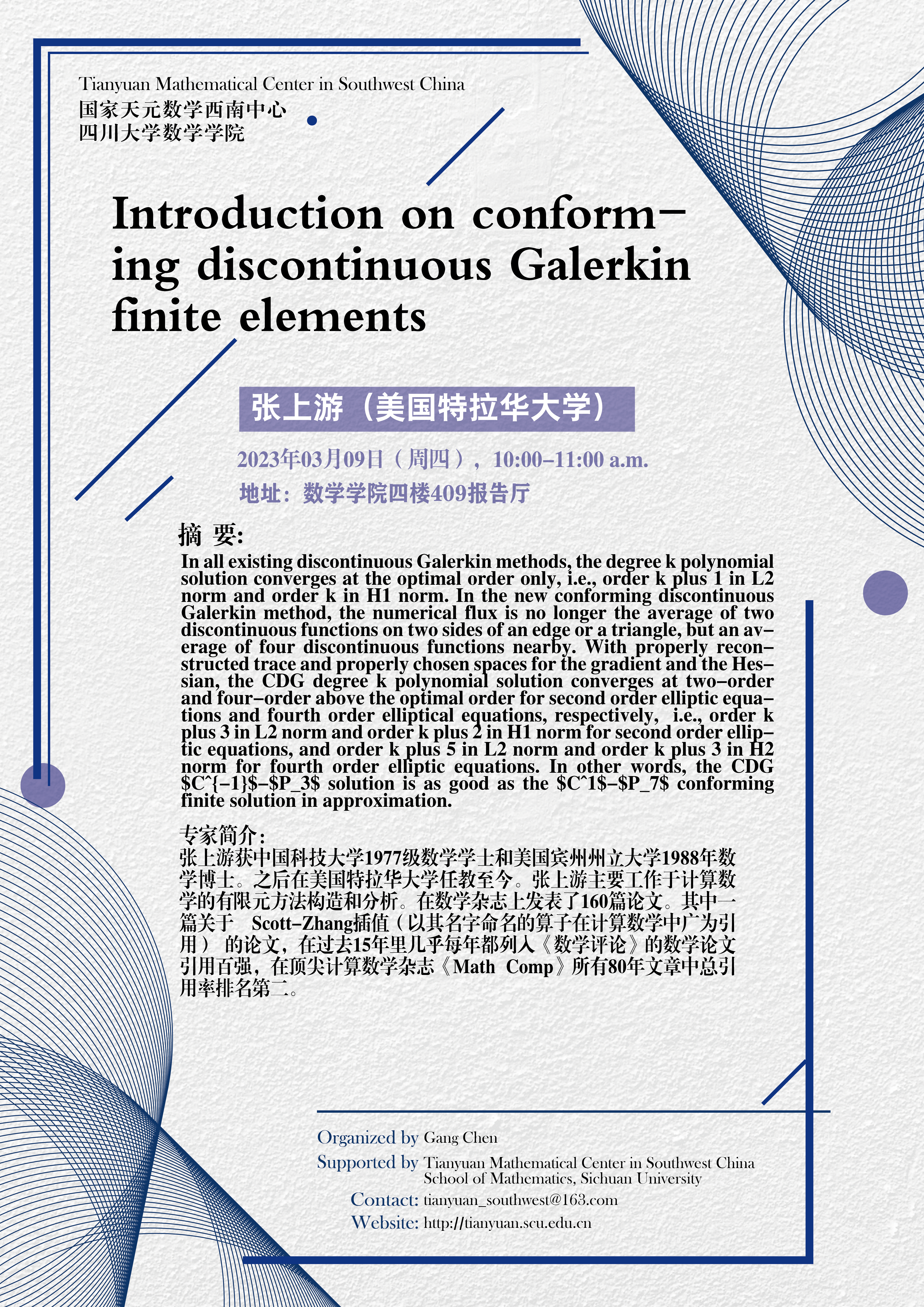Introduction on conforming discontinuous Galerkin finite elements

In all existing discontinuous Galerkin methods, the degree k polynomial solution converges at the optimal order only, i.e., order k plus 1 in L2 norm and order k in H1 norm. In the new conforming discontinuous Galerkin method, the numerical flux is no longer the average of two discontinuous functions on two sides of an edge or a triangle, but an average of four discontinuous functions nearby. With properly reconstructed trace and properly chosen spaces for the gradient and the Hessian, the CDG degree k polynomial solution converges at two-order and four-order above the optimal order for second order elliptic equations and fourth order elliptical equations, respectively,  i.e., order k plus 3 in L2 norm and order k plus 2 in H1 norm for second order elliptic equations, and order k plus 5 in L2 norm and order k plus 3 in H2 norm for fourth order elliptic equations. In other words, the CDG \$C^{-1}\$-\$P_3\$ solution is as good as the \$C^1\$-\$P_7\$ conforming finite solution in approximation.